# 金庸的武侠世界

• 《飞狐外传》（1960年）
• 《雪山飞狐》（1959年）
• 《连城诀》（1963年）
• 《天龙八部》（1963年）
• 《射雕英雄传》（1957年）
• 《白马啸西风》（1961年）
• 《鹿鼎记》（1969年）
• 《笑傲江湖》（1967年）
• 《书剑恩仇录》（1955年）
• 《神雕侠侣》（1959年）
• 《侠客行》（1965年）
• 《倚天屠龙记》（1961年）
• 《碧血剑》（1956年）
• 《鸳鸯刀》（1961年）
• 《越女剑》（1970年）

## 准备工作

In :

from __future__ import unicode_literals



In :

import numpy as np
import scipy as sp
import matplotlib.pyplot as plt
%matplotlib inline

x = range(10)
plt.plot(x)
plt.title("中文")
plt.show()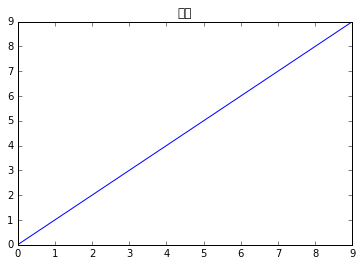Windows 7 及以上的系统中，字体位置为 C:/Windows/Fonts，例如：

• 宋体：C:/Windows/Fonts/simsum.ttc

Linux 系统可以通过 fc-list 命令查看已有的字体和相应的位置，例如：

• /usr/share/fonts/truetype/osx-font-family/Songti.ttc: Songti TC,宋體\-繁,宋体\-繁:style=Bold,粗體,粗体
• /usr/share/fonts/truetype/osx-font-family/Devanagari Sangam MN.ttc: Devanagari Sangam MN,देवनागरी संगम एम॰एन॰:style=Bold,粗體,Fed,Fett,Puolilihava,Gras,Grassetto,ボールド,볼드체,Vet,Fet,Negrito,Жирный,बोल्ड,粗体,Negrita
• /usr/share/fonts/truetype/osx-font-family/Iowan Old Style.ttc: Iowan Old Style,Iowan Old Style Black:style=Black Italic,Italic

• 比如 Yahei Consolas 的字体 YaHei.Consolas.1.11b.ttf

font_xxx = FontProperties(fname="/usr/share/fonts/truetype/osx-font-family/Songti.ttc")
font_xxx = FontProperties(fname="C://Windows//Fonts//simsum.ttc")


• simsum.ttc
• YaHei.Consolas.1.11b.ttf

In :

from matplotlib.font_manager import FontProperties

font_simsum = FontProperties(fname="simsun.ttc")
font_yahei_consolas = FontProperties(fname="YaHei.Consolas.1.11b.ttf")



In :

x = range(10)
plt.plot(x)
plt.title("中文", fontproperties=font_yahei_consolas, fontsize=14)
plt.show()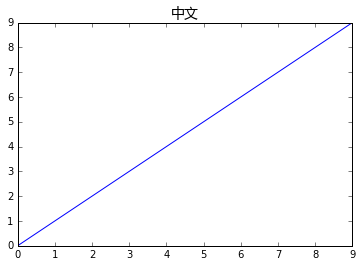## 小说概览

In :

!ls novels


书剑恩仇录.txt   天龙八部.txt    碧血剑.txt    越女剑.txt    飞狐外传.txt



In :

with open('novels/鸳鸯刀.txt') as f:
# 去掉结尾的换行符
data = [line.strip().decode('utf8') for line in f.readlines()]

for line in data[:13]:
if line.strip():
print line



鸳鸯刀
四个劲装结束的汉子并肩而立，拦在当路！
若是黑道上山寨的强人，不会只有四个，莫非在这黑沉沉的松林之中，暗中还埋伏下大批人手？如是剪径的小贼，见了这么声势浩大的镖队，远避之唯恐不及，哪敢这般大模大样的拦路挡道？难道竟是武林高手，冲着自己而来？
凝神打量四人：最左一人短小精悍，下巴尖削，手中拿着一对峨嵋钢刺。第二个又高又肥，便如是一座铁塔摆在地下，身前放着一块大石碑，碑上写的是“先考黄府君诚本之墓”，这自是一块墓碑了，不知放在身前有何用意？黄诚本？没听说江湖上有这么一位前辈高手啊！第三个中等身材，白净脸皮，若不是一副牙齿向外凸出了一寸，一个鼻头低陷了半寸，倒算得上是一位相貌英俊的人物，他手中拿的是一对流星锤。最右边的是个病夫模样的中年人，衣衫褴褛，咬着一根旱烟管，双目似睁似闭，嘴里慢慢喷着烟雾，竟是没将这一队七十来人的镖队瞧在眼里。
那三人倒还罢了，这病夫定是个内功深湛的劲敌。顷刻之间，江湖上许多轶闻往事涌上了心头：一个白发婆婆空手杀死了五名镖头，劫走了一支大镖；一个老乞丐大闹太原府公堂，割去了知府的首级，倏然间不知去向；一个美貌大姑娘打倒了晋北大同府享名二十余年的张大拳师……越是貌不惊人、满不在乎的人物，越是武功了得，江湖上有言道：“真人不露相，露相不真人。”
瞧着这个闭目抽烟的病夫，陕西西安府威信镖局的总镖头、“铁鞭镇八方”周威信不由得深自踌躇起来，不由自主的伸手去摸了一摸背上的包袱。



## 出场人物

小说1

……


In :

with open('names.txt') as f:
# 去掉结尾的换行符
data = [line.strip().decode('utf8') for line in f.readlines()]

novels = data[::2]
names = data[1::2]

novel_names = {k: v.split() for k, v in zip(novels, names)}

for name in novel_names['天龙八部'][:20]:
print name


刀白凤



## 寻找主角光环

In :

def find_main_charecters(novel, num=10):
with open('novels/{}.txt'.format(novel)) as f:
count = []
for name in novel_names[novel]:
count.append([name, data.count(name)])
count.sort(key=lambda x: x)
_, ax = plt.subplots()

numbers = [x for x in count[-num:]]
names = [x for x in count[-num:]]
ax.barh(range(num), numbers, color='red', align='center')
ax.set_title(novel, fontsize=14, fontproperties=font_yahei_consolas)
ax.set_yticks(range(num))
ax.set_yticklabels(names, fontsize=14, fontproperties=font_yahei_consolas)



In :

find_main_charecters("天龙八部")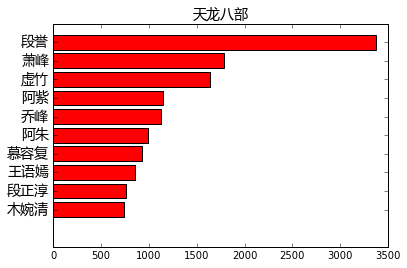In :

find_main_charecters("射雕英雄传")
find_main_charecters("神雕侠侣")
find_main_charecters("倚天屠龙记")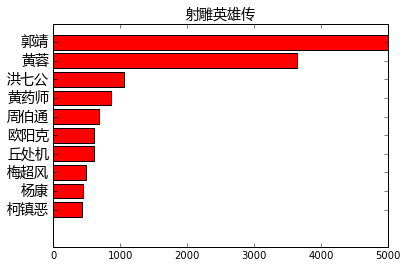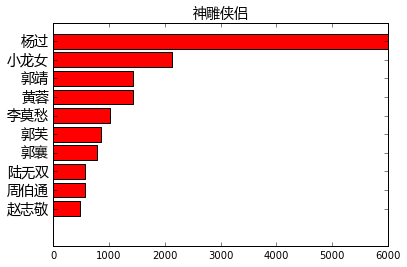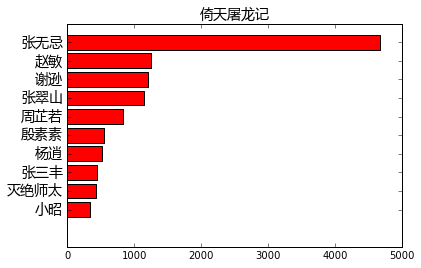## Word2Vec

Word2Vec 是一款将词表征为实数值向量的高效工具，原理就不过多介绍了，感兴趣的可以自行搜索，

gensim 包提供了一个 Python 版的实现。

pip install gensim


In :

import gensim


Using gpu device 1: Tesla C2075 (CNMeM is disabled, CuDNN not available)



## 中文分词

pip install jieba


In :

import jieba



jieba 包具有识别新词的能力，不过为了得到更准确的分词结果，我们可以将人名导入 jieba 库的字典，除此之外，我们还加入门派和武功的专有名词：

In :

for _, names in novel_names.iteritems():
for name in names:

with open("kungfu.txt") as f:
kungfu_names = [line.decode('utf8').strip() for line in f.readlines()]

with open("bangs.txt") as f:
bang_names = [line.decode('utf8').strip() for line in f.readlines()]

for name in kungfu_names:

for name in bang_names:


Building prefix dict from the default dictionary ...
DEBUG:jieba:Building prefix dict from the default dictionary ...
Prefix dict has been built succesfully.
DEBUG:jieba:Prefix dict has been built succesfully.



In :

novels = ["书剑恩仇录",
"天龙八部",
"碧血剑",
"越女剑",
"飞狐外传",
"侠客行",
"射雕英雄传",
"神雕侠侣",
"连城诀",
"鸳鸯刀",
"倚天屠龙记",
"白马啸西风",
"笑傲江湖",
"雪山飞狐",
"鹿鼎记"]

sentences = []

for novel in novels:
print "处理：{}".format(novel)
with open('novels/{}.txt'.format(novel)) as f:
data = [line.decode('utf8').strip() for line in f.readlines() if line.decode('utf8').strip()]
for line in data:
words = list(jieba.cut(line))
sentences.append(words)


处理：书剑恩仇录



## 训练模型

In :

model = gensim.models.Word2Vec(sentences, size=100, window=5, min_count=5, workers=4)



In :

model.save("louis_cha.model")



In :

model = gensim.models.Word2Vec.load("louis_cha.model")



## 相似度结果

In :

for k, s in model.most_similar(positive=["乔峰", "萧峰"]):
print k, s


段正淳 0.839533925056



In :

for k, s in model.most_similar(positive=["阿朱"]):
print k, s


钟灵 0.789930582047



In :

for k, s in model.most_similar(positive=["丐帮"]):
print k, s


长乐帮 0.807791054249



In :

for k, s in model.most_similar(positive=["降龙十八掌"]):
print k, s


打狗棒法 0.89123404026



In :

def find_relationship(a, b, c):
"""
返回 d
a与b的关系，跟c与d的关系一样
"""
d, _ = model.most_similar(positive=[c, b], negative=[a])
print "给定“{}”与“{}”，“{}”和“{}”有类似的关系".format(a, b, c, d)

find_relationship("段誉", "段公子", "乔峰")


给定“段誉”与“段公子”，“乔峰”和“乔帮主”有类似的关系



In :

# 情侣对
find_relationship("郭靖", "黄蓉", "杨过")

# 岳父女婿
find_relationship("令狐冲", "任我行", "郭靖")

# 非情侣
find_relationship("郭靖", "华筝", "杨过")


给定“郭靖”与“黄蓉”，“杨过”和“小龙女”有类似的关系



In :

# 韦小宝
find_relationship("杨过", "小龙女", "韦小宝")
find_relationship("令狐冲", "盈盈", "韦小宝")
find_relationship("张无忌", "赵敏", "韦小宝")


给定“杨过”与“小龙女”，“韦小宝”和“康熙”有类似的关系



In :

find_relationship("郭靖", "降龙十八掌", "黄蓉")
find_relationship("武当", "张三丰", "少林")
find_relationship("任我行", "魔教", "令狐冲")


给定“郭靖”与“降龙十八掌”，“黄蓉”和“打狗棒法”有类似的关系



## 人物聚类分析

In :

all_names = []
word_vectors = None

for name in novel_names["天龙八部"]:
if name in model:
all_names.append(name)
if word_vectors is None:
word_vectors = model[name]
else:
word_vectors = np.vstack((word_vectors, model[name]))

all_names = np.array(all_names)



### KMeans

In :

from sklearn.cluster import KMeans



In :

N = 3
label = KMeans(N).fit(word_vectors).labels_

for c in range(N):
print "\n类别{}：".format(c+1)
for idx, name in enumerate(all_names[label==c]):
print name,
if idx % 10 == 9:
print
print


类别1：



In :

N = 4
c = sp.stats.mode(label).mode
remain_names = all_names[label!=c]
remain_vectors = word_vectors[label!=c]

remain_label = KMeans(N).fit(remain_vectors).labels_

for c in range(N):
print "\n类别{}：".format(c+1)
for idx, name in enumerate(remain_names[remain_label==c]):
print name,
if idx % 10 == 9:
print
print


类别1：



### 层级聚类

In :

all_names = []
word_vectors = None

for name in novel_names["倚天屠龙记"]:
if name in model:
all_names.append(name)
if word_vectors is None:
word_vectors = model[name]
else:
word_vectors = np.vstack((word_vectors, model[name]))

all_names = np.array(all_names)



In :

import scipy.cluster.hierarchy as sch



In :

Y = sch.linkage(word_vectors, method="ward")

_, ax = plt.subplots(figsize=(10, 40))

Z = sch.dendrogram(Y, orientation='right')
idx = Z['leaves']

ax.set_xticks([])
ax.set_yticklabels(all_names[idx], fontproperties=font_yahei_consolas)
ax.set_frame_on(False)

plt.show()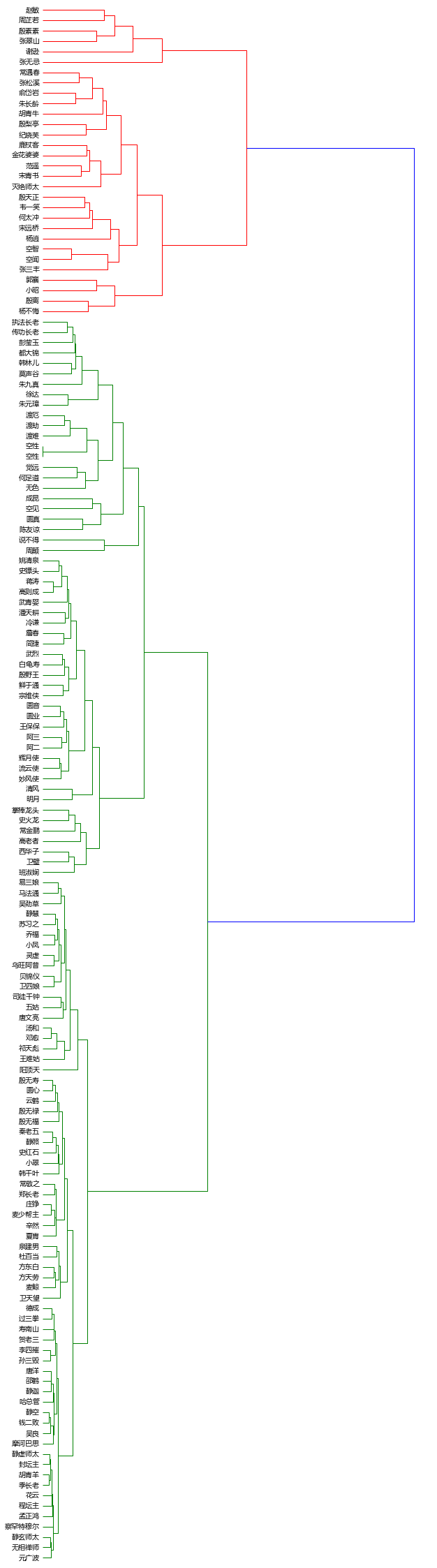### 门派和武功

In :

all_names = []
word_vectors = None
for name in kungfu_names:
if name in model:
all_names.append(name)
if word_vectors is None:
word_vectors = model[name]
else:
word_vectors = np.vstack((word_vectors, model[name]))

all_names = np.array(all_names)

_, ax = plt.subplots(figsize=(10, 35))

Z = sch.dendrogram(Y, orientation='right')
idx = Z['leaves']

ax.set_xticks([])
ax.set_yticklabels(all_names[idx], fontproperties=font_yahei_consolas)
ax.set_frame_on(False)

plt.show()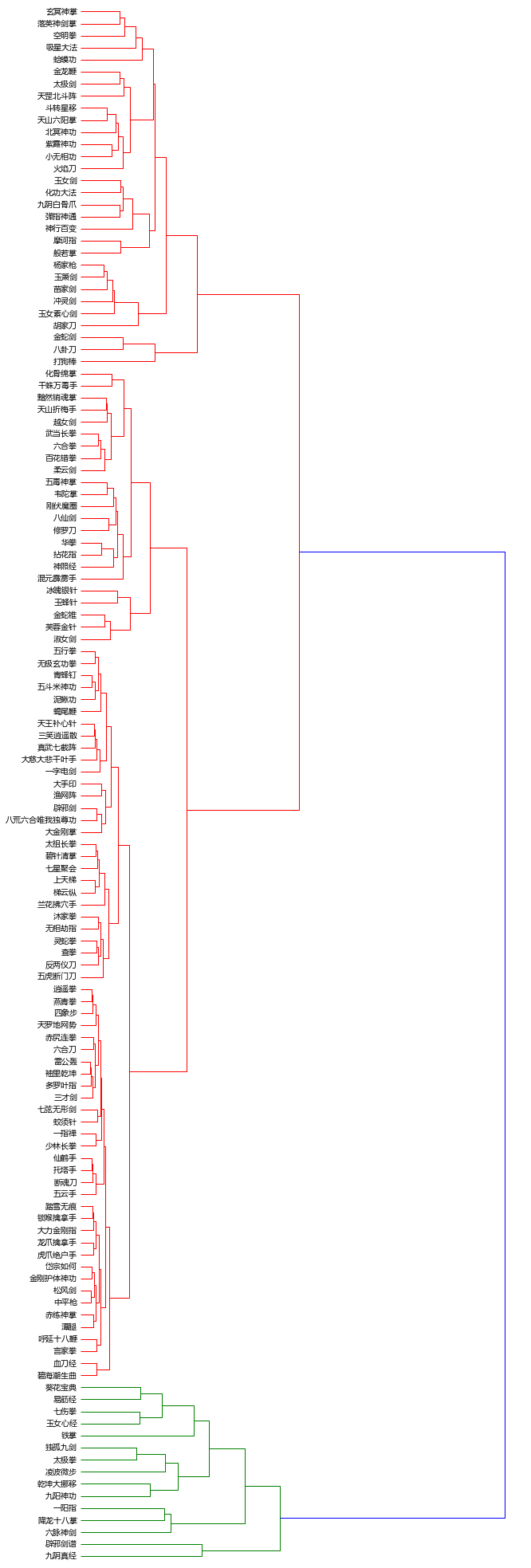In :

all_names = []
word_vectors = None
for name in bang_names:
if name in model:
all_names.append(name)
if word_vectors is None:
word_vectors = model[name]
else:
word_vectors = np.vstack((word_vectors, model[name]))

all_names = np.array(all_names)

_, ax = plt.subplots(figsize=(10, 25))

Z = sch.dendrogram(Y, orientation='right')
idx = Z['leaves']

ax.set_xticks([])
ax.set_yticklabels(all_names[idx], fontproperties=font_yahei_consolas)
ax.set_frame_on(False)

plt.show()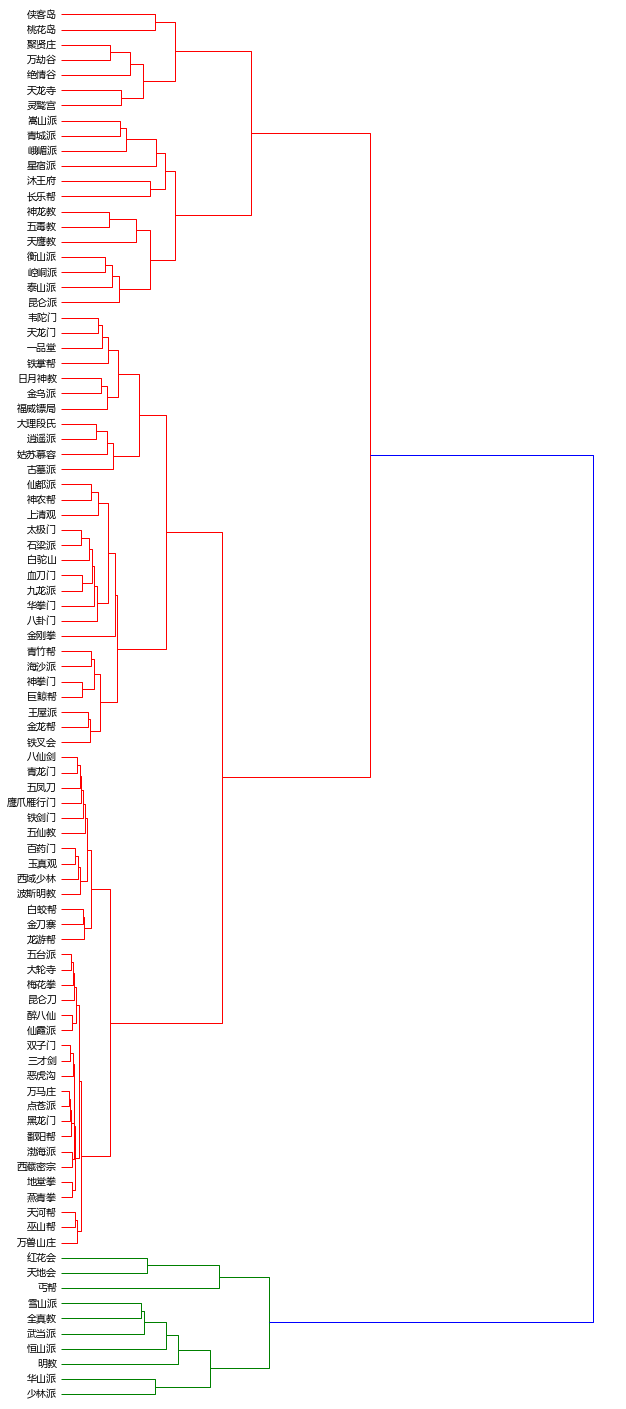## 总结